1. /
2. CBSE
3. /
4. Class 06
5. /
6. Mathematics
7. /
8. NCERT Solutions for Class...

# NCERT Solutions for Class 6 Maths Exercise 7.4

NCERT solutions for Maths Fractions## NCERT Solutions for Class 6 Maths Fractions

###### Question 1.Write shaded portion as fraction. Arrange them in ascending and descending order using correct sign ‘<’, ‘>’, ‘=’ between the fractions:

(a)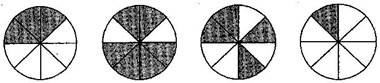(b)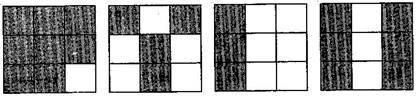(c)

Show {tex}\frac{2}{6},\frac{4}{6},\frac{8}{6}{/tex} and {tex}\frac{6}{6}{/tex} on the number line. Put appropriate signs between the fractions given:

{tex}\frac{5}{6}\boxed{{\text{ }}}\frac{2}{6},{/tex} {tex}\frac{3}{6}\boxed{{\text{ }}}0,{/tex}

{tex}\frac{1}{6}\boxed{{\text{ }}}\frac{6}{6},{/tex} {tex}\frac{8}{6}\boxed{{\text{ }}}\frac{5}{6}{/tex}

(a) {tex}\frac{3}{8},\frac{6}{8},\frac{4}{8},\frac{1}{8}{/tex}

Ascending order: {tex}\frac{1}{8} < \frac{3}{8} < \frac{4}{8} < \frac{6}{8}{/tex}

Descending order: {tex}\frac{6}{8} > \frac{4}{8} > \frac{3}{8} > \frac{1}{8}{/tex}

(b) {tex}\frac{8}{9},\frac{4}{9},\frac{3}{9},\frac{6}{9}{/tex}

Ascending order: {tex}\frac{3}{9} < \frac{4}{9} < \frac{6}{9} < \frac{8}{9}{/tex}

Descending order: {tex}\frac{8}{9} > \frac{6}{9} > \frac{4}{9} > \frac{3}{9}{/tex}

(c) Number line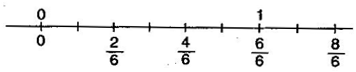{tex}\frac{5}{6}\boxed > \frac{2}{6}{/tex} {tex}\frac{1}{6}\boxed < \frac{6}{6}{/tex}

{tex}\frac{3}{6}\boxed > \frac{0}{6}{/tex} {tex}\frac{8}{6}\boxed > \frac{5}{6}{/tex}

###### Question 2.Compare the fractions and put an appropriate sign:

(a) {tex}\frac{3}{6}\boxed{{\text{ }}}\frac{5}{6}{/tex}

(b) {tex}\frac{1}{7}\boxed{{\text{ }}}\frac{1}{4}{/tex}

(c) {tex}\frac{4}{5}\boxed{{\text{ }}}\frac{5}{5}{/tex}

(d) {tex}\frac{3}{5}\boxed{{\text{ }}}\frac{3}{7}{/tex}

(a) {tex}\frac{3}{6}\boxed < \frac{5}{6}{/tex}

(b) {tex}\frac{1}{7}\boxed < \frac{1}{4}{/tex}

(c) {tex}\frac{4}{5}\boxed < \frac{5}{5}{/tex}

(d) {tex}\frac{3}{5}\boxed > \frac{3}{7}{/tex}

###### NCERT Solutions for Class 6 Maths Exercise 7.4

Question 3.Make five more each pairs and put appropriate signs.

(a) {tex}\frac{9}{{10}}\boxed > \frac{6}{{10}}{/tex}

(b) {tex}\frac{1}{3}\boxed > \frac{1}{6}{/tex}

(c) {tex}\frac{1}{8}\boxed < \frac{1}{5}{/tex}

(d) {tex}\frac{7}{8}\boxed < \frac{{11}}{8}{/tex}

(e) {tex}\frac{{11}}{{13}}\boxed > \frac{9}{{13}}{/tex}

###### Question 4.Look at the figures and write ‘<’ or ‘>’ between the given pairs of fractions: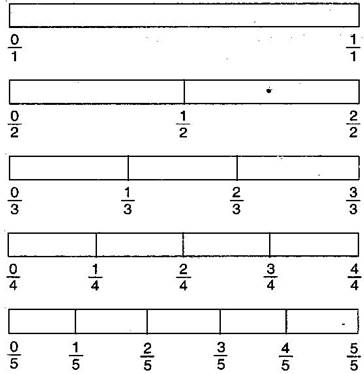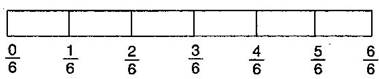(a) {tex}\frac{1}{6}\boxed{{\text{ }}}\frac{1}{3}{/tex}

(b) {tex}\frac{3}{4}\boxed{{\text{ }}}\frac{2}{6}{/tex}

(c) {tex}\frac{2}{3}\boxed{{\text{ }}}\frac{2}{4}{/tex}

(d) {tex}\frac{6}{6}\boxed{{\text{ }}}\frac{3}{3}{/tex}

(e) {tex}\frac{5}{6}\boxed{{\text{ }}}\frac{5}{5}{/tex}

Make five more such problems and solve them with your friends.

(a) {tex}\frac{1}{6}\boxed < \frac{1}{3}{/tex}

(b) {tex}\frac{3}{4}\boxed > \frac{2}{6}{/tex}

(c) {tex}\frac{2}{3}\boxed > \frac{2}{4}{/tex}

(d) {tex}\frac{6}{6}\boxed = \frac{3}{3}{/tex}

(e) {tex}\frac{5}{6}\boxed < \frac{5}{5}{/tex}

Five more such problems:

(a) {tex}\frac{1}{2}\boxed{{\text{ }}}\frac{3}{6}{/tex}

(b) {tex}\frac{2}{3}\boxed{{\text{ }}}\frac{3}{5}{/tex}

(c) {tex}\frac{3}{4}\boxed{{\text{ }}}\frac{4}{6}{/tex}

(d) {tex}\frac{5}{6}\boxed{{\text{ }}}\frac{2}{2}{/tex}

(e) {tex}\frac{0}{1}\boxed{{\text{ }}}\frac{0}{6}{/tex}

Sol.

(a) {tex}\frac{1}{2}\boxed{{\text{ = }}}\frac{3}{6}{/tex}

(b) {tex}\frac{2}{3}\boxed{{\text{ > }}}\frac{3}{5}{/tex}

(c) {tex}\frac{3}{4}\boxed{{\text{ > }}}\frac{4}{6}{/tex}

(d) {tex}\frac{5}{6}\boxed{{\text{ < }}}\frac{2}{2}{/tex}

(e) {tex}\frac{0}{1}\boxed{{\text{ = }}}\frac{0}{6}{/tex}

NCERT Solutions for Class 6 Maths Exercise 7.4

###### Question 5.How quickly can you do this? Fill appropriate sign (<, =, >):

(a) {tex}\frac{1}{2}\boxed{{\text{ }}}\frac{1}{5}{/tex}

(b) {tex}\frac{2}{4}\boxed{{\text{ }}}\frac{3}{6}{/tex}

(c) {tex}\frac{3}{5}\boxed{{\text{ }}}\frac{2}{3}{/tex}

(d) {tex}\frac{3}{4}\boxed{{\text{ }}}\frac{2}{8}{/tex}

(e) {tex}\frac{3}{5}\boxed{{\text{ }}}\frac{6}{5}{/tex}

(f) {tex}\frac{7}{9}\boxed{{\text{ }}}\frac{3}{9}{/tex}

(g) {tex}\frac{1}{4}\boxed{{\text{ }}}\frac{2}{8}{/tex}

(h) {tex}\frac{6}{{10}}\boxed{{\text{ }}}\frac{4}{5}{/tex}

(i) {tex}\frac{3}{4}\boxed{{\text{ }}}\frac{7}{8}{/tex}

(j) {tex}\frac{6}{{10}}\boxed{{\text{ }}}\frac{4}{5}{/tex}

(k) {tex}\frac{5}{7}\boxed{{\text{ }}}\frac{{15}}{{21}}{/tex}

(a) {tex}\frac{1}{2}\boxed{{\text{ > }}}\frac{1}{5}{/tex}

(b) {tex}\frac{2}{4}\boxed{{\text{ = }}}\frac{3}{6}{/tex}

(c) {tex}\frac{3}{5}\boxed{{\text{ < }}}\frac{2}{3}{/tex}

(d) {tex}\frac{3}{4}\boxed{{\text{ > }}}\frac{2}{8}{/tex}

(e) {tex}\frac{3}{5}\boxed{{\text{ < }}}\frac{6}{5}{/tex}

(f) {tex}\frac{7}{9}\boxed{{\text{ > }}}\frac{3}{9}{/tex}

(g) {tex}\frac{1}{4}\boxed{{\text{ = }}}\frac{2}{8}{/tex}

(h) {tex}\frac{6}{{10}}\boxed{{\text{ < }}}\frac{4}{5}{/tex}

(i) {tex}\frac{3}{4}\boxed{{\text{ < }}}\frac{7}{8}{/tex}

(j) {tex}\frac{6}{{10}}\boxed{{\text{ < }}}\frac{4}{5}{/tex}

(k) {tex}\frac{5}{7}\boxed{{\text{ = }}}\frac{{15}}{{21}}{/tex}

###### Question 6.The following fractions represent just three different numbers. Separate them into three groups of equivalent fractions, by changing each one to its simplest form:

(a) {tex}\frac{2}{{12}}{/tex}

(b) {tex}\frac{3}{{15}}{/tex}

(c) {tex}\frac{8}{{50}}{/tex}

(d) {tex}\frac{{16}}{{100}}{/tex}

(e) {tex}\frac{{10}}{{60}}{/tex}

(f) {tex}\frac{{15}}{{75}}{/tex}

(g) {tex}\frac{{12}}{{60}}{/tex}

(h) {tex}\frac{{16}}{{96}}{/tex}

(i) {tex}\frac{{12}}{{75}}{/tex}

(j) {tex}\frac{{12}}{{72}}{/tex}

(k) {tex}\frac{3}{{18}}{/tex}

(l) {tex}\frac{4}{{25}}{/tex}

(a) {tex}\frac{2}{12}=\frac{1}{6}{/tex}

(b) {tex}\frac{3}{15}=\frac{1}{5}{/tex}

(c) {tex}\frac{8}{50}=\frac{4}{25}{/tex}

(d) {tex}\frac{16}{100}=\frac{4}{25}{/tex}

(e) {tex}\frac{10}{60}=\frac{1}{6}{/tex}

(f) {tex}\frac{15}{75}=\frac{1}{5}{/tex}

(g) {tex}\frac{12}{60}=\frac{1}{5}{/tex}

(h) {tex}\frac{16}{96}=\frac{1}{6}{/tex}

(i) {tex}\frac{12}{75}=\frac{4}{25}{/tex}

(j) {tex}\frac{12}{72}=\frac{1}{6}{/tex}

(k) {tex}\frac{3}{18}=\frac{1}{6}{/tex}

(l) {tex}\frac{4}{25}=\frac{4}{25}{/tex}

Equivalent groups:

I group: {tex}\frac{1}{5}{/tex} [(b), (f), (g)]

II group: {tex}\frac{1}{6}{/tex} [(a), (e), (h), (j), (k)]

III group: {tex}\frac{4}{{25}}{/tex} [(c), (d), (i), (l)]

NCERT Solutions for Class 6 Maths Exercise 7.4

###### Question 7.Find answers to the following. Write and indicate how you solved them:

(a) Is {tex}\frac{5}{9}{/tex} equal to {tex}\frac{4}{5}{/tex} ?

(b) Is {tex}\frac{9}{{16}}{/tex} equal to {tex}\frac{5}{9}{/tex} ?

(c) Is {tex}\frac{4}{5}{/tex} equal to {tex}\frac{{16}}{{20}}{/tex} ?

(d) Is {tex}\frac{1}{{15}}{/tex} equal to {tex}\frac{4}{{30}}{/tex} ?

(a){tex}\frac{5}{9}{/tex} and {tex}\frac{4}{5}{/tex}

{tex} \Rightarrow {/tex} {tex}\frac{{5 \times 5}}{{9 \times 5}} = \frac{{25}}{{45}}{/tex} and {tex}\frac{{4 \times 9}}{{5 \times 9}} = \frac{{36}}{{45}}{/tex} [{tex}\because {/tex} L.C.M. of 9 and 5 is 45]

Since, {tex}\frac{{25}}{{45}} \ne \frac{{36}}{{45}}{/tex}

Therefore, {tex}\frac{5}{9} \ne \frac{4}{5}{/tex}

(b) {tex}\frac{9}{{16}}{/tex} and {tex}\frac{5}{9}{/tex}

{tex} \Rightarrow {/tex}{tex}\frac{{9 \times 9}}{{16 \times 9}} = \frac{{81}}{{144}}{/tex} and {tex}\frac{{5 \times 16}}{{9 \times 16}} = \frac{{80}}{{144}}{/tex} [{tex}\because {/tex} L.C.M. of 16 and 9 is 144]

Since, {tex}\frac{{81}}{{144}} \ne \frac{{80}}{{144}}{/tex}

Therefore, {tex}\frac{9}{{16}} \ne \frac{5}{9}{/tex}

(c) {tex}\frac{4}{5}{/tex} and {tex}\frac{{16}}{{20}}{/tex}

{tex} \Rightarrow {/tex}{tex}\frac{{4 \times 20}}{{5 \times 20}} = \frac{{80}}{{100}}{/tex} and {tex}\frac{{16 \times 5}}{{20 \times 5}} = \frac{{80}}{{100}}{/tex} [{tex}\because {/tex} L.C.M. of 5 and 20 is 100]

Since, {tex}\frac{{80}}{{100}} = \frac{{80}}{{100}}{/tex}

Therefore, {tex}\frac{4}{5} = \frac{{16}}{{20}}{/tex}

(d) {tex}\frac{1}{{15}}{/tex} and {tex}\frac{4}{{30}}{/tex}

{tex}\Rightarrow {/tex}{tex}\frac{{1 \times 2}}{{15 \times 2}} = \frac{2}{{30}}{/tex} and {tex}\frac{{4 \times 1}}{{30 \times 1}} = \frac{4}{{30}}{/tex} [{tex}\because {/tex} L.C.M. of 15 and 30 is 30]

Since, {tex}\frac{4}{{30}} = \frac{4}{{30}}{/tex} Therefore,

{tex}\frac{1}{{15}} = \frac{4}{{30}}{/tex}

NCERT Solutions for Class 6 Maths Exercise 7.4

###### Question 8.Ila read 25 pages of a book containing 100 pages. Lalita read {tex}\frac{2}{5}{/tex} of the same book. Who read less?

Ila read 25 pages out of 100 pages.

Fraction of reading the pages = {tex}\frac{{25}}{{100}} = \frac{1}{4}th{/tex} part of book

Lalita read {tex}\frac{2}{5}th{/tex} part of book = {tex}\frac{{40}}{{100}}{/tex} pages

Since {tex}\frac{1}{4} < \frac{2}{5}{/tex}

###### Question 9.Rafiq exercised for {tex}\frac{3}{6}{/tex} of an hour, while Rohit exercised for {tex}\frac{3}{4}{/tex} of an hour. Who exercised for a longer time?

Rafiq exercised {tex}\frac{3}{6}{/tex} of an hour.

Rohit exercised {tex}\frac{3}{4}{/tex} of an hour.

Since {tex}\frac{3}{4} > \frac{3}{6}{/tex}

Therefore, Rohit exercised for a longer time.

NCERT Solutions for Class 6 Maths Exercise 7.4

###### Question 10.In a class A of 25 students, 20 passed in first class; in another class B of 30 students, 24 passed in first class. In which class was a greater fraction of students getting first class?

In class A, 20 passed out of 25, i.e., {tex}\frac{{20}}{{25}}{/tex} = {tex}\frac{4}{5}{/tex}

In class B, 24 passed out of 30, i.e., {tex}\frac{{24}}{{30}}{/tex} = {tex}\frac{4}{5}{/tex}

Hence, each class have same fraction of student getting first class.

## NCERT Solutions for Class 6 Maths Exercise 7.4

NCERT Solutions Class 6 Maths PDF (Download) Free from myCBSEguide app and myCBSEguide website. Ncert solution class 6 Maths includes text book solutions from Class 6 Maths Book . NCERT Solutions for CBSE Class 6 Maths have total 14 chapters. 6 Maths NCERT Solutions in PDF for free Download on our website. Ncert Maths class 6 solutions PDF and Maths ncert class 6 PDF solutions with latest modifications and as per the latest CBSE syllabus are only available in myCBSEguide.

## CBSE app for Students

To download NCERT Solutions for Class 6 Maths, Social Science Computer Science, Home Science, Hindi English, Maths Science do check myCBSEguide app or website. myCBSEguide provides sample papers with solution, test papers for chapter-wise practice, NCERT solutions, NCERT Exemplar solutions, quick revision notes for ready reference, CBSE guess papers and CBSE important question papers. Sample Paper all are made available through the best app for CBSE students and myCBSEguide website.### Test Generator

Create question paper PDF and online tests with your own name & logo in minutes.### myCBSEguide

Question Bank, Mock Tests, Exam Papers, NCERT Solutions, Sample Papers, Notes

### 1 thought on “NCERT Solutions for Class 6 Maths Exercise 7.4”

1. Questions are not correct